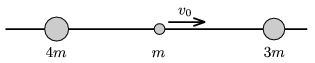Mathematical and Physical Journal
for High Schools
Issued by the MATFUND Foundation
 Already signed up? New to KöMaL?

#Problem P. 4208. (December 2009)

P. 4208. Three marbles of mass 4m, m and 3m can slide without friction along a horizontal rod. (There are holes in the balls.) At a certain moment the middle marble is given an initial speed of v0=5 m/s towards the right. After the totally elastic collisions determine the

a) velocities of the marbles;

b) the ratio of the kinetic energies of the marbles.(4 pont)

Deadline expired on January 11, 2010.

Sorry, the solution is available only in Hungarian. Google translation

Megoldás. $\displaystyle a)$ A bal oldali test balra halad 1 m/s, a középső jobbra 1,5 m/s sebességgel, a jobb oldali jobbra 2,5 m/s sebességgel.

$\displaystyle b)$ A mozgási energiák aránya: $\displaystyle E_{4m}:E_{m}:E_{3m}= 14:9:75$.

### Statistics:

 156 students sent a solution. 4 points: 122 students. 3 points: 8 students. 2 points: 7 students. 1 point: 8 students. 0 point: 9 students. Unfair, not evaluated: 2 solutionss.

Problems in Physics of KöMaL, December 2009